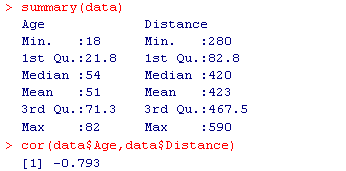## Linear Regression (4 of 4)

### Learning Objectives

• For a linear relationship, use the least squares regression line to model the pattern in the data and to make predictions.

In the previous activity we used technology to find the least-squares regression line from the data values.

We can also find the equation for the least-squares regression line from summary statistics for x and y and the correlation.

If we know the mean and standard deviation for x and y, along with the correlation (r), we can calculate the slope b and the starting value a with the following formulas:

$b=\frac{r⋅{s}_{y}}{{s}_{x}}\text{ and }a=\stackrel{¯}{y}-b\stackrel{¯}{x}$

As before, the equation of the linear regression line is

Predicted y = a + b * x

### Example: Highway Sign Visibility

We will now find the equation of the least-squares regression line using the output from a statistics package.• The slope of the line is $b=\left(-0.793\right)\ast \left(\frac{82.8}{21.78}\right)=-3$
• The intercept of the line is a = 423 – (-3 * 51) = 576 and therefore the least-squares regression line for this example is Predicted distance = 576 + (-3 * Age), which can also be written as Predicted distance = 576 – 3 * Age

### Learn By Doing

Now you know how to calculate the least-squares regression line from the correlation and the mean and standard deviation of x and y. But what do these formulas tell us about the least-squares line?

We know that the intercept a is the predicted value when x = 0.

The formula $a=\stackrel{¯}{y}\text{}\text{−}\text{}b⋅\stackrel{¯}{x}$ tells us that the we can find the intercept using the point: ($\overline{x},\overline{y}$).

This is interesting because it says that every least-squares regression line contains this point. In other words, the least-squares regression line goes through the mean of x and the mean of y.

We also know that the slope of the least-squares regression line is the average change in the predicted response when the explanatory variable increases by 1 unit.

The slope formula

$b=\frac{r⋅{s}_{y}}{{s}_{x}}$

tells us that the slope is related to the correlation in this way: when x increases an x standard deviation, the predicted y-value does not change by a y standard deviation. Instead, the predicted y-value changes by less than a y standard deviation. The change is a fraction of a y standard deviation, and that fraction is r. Another way to say this is that when x increases by a standard deviation in x, the average change in the predicted response is a fractional change of r standard deviations in y.

It is not surprising that slope and correlation are connected. We already know that when a linear relationship is positive, the correlation and the slope are positive. Similarly, when a linear relationship is negative, the correlation and slope are both negative. But now we understand this connection more precisely.

### Let’s Summarize

• The line that best summarizes a linear relationship is the least-squares regression line. The least-squares line is the best fit for the data because it gives the best predictions with the least amount of overall error. The most common measurement of overall error is the sum of the squares of the errors (SSE). The least-squares line is the line with the smallest SSE.
• We use the least-squares regression line to predict the value of the response variable from a value of the explanatory variable.
• Prediction for values of the explanatory variable that fall outside the range of the data is called extrapolation. These predictions are unreliable because we do not know if the pattern observed in the data continues outside the range of the data. Avoid making predictions outside the range of the data.
• The slope of the least-squares regression line is the average change in the predicted values of the response variable when the explanatory variable increases by 1 unit.
• We have two methods for finding the equation of the least-squares regression line:

Predicted y = a + b * x

Method 1: We use technology to find the equation of the least-squares regression line:

Predicted y = a + b * x

Method 2: We use summary statistics for x and y and the correlation. In this method we can calculate the slope b and the y-intercept a using the following:

$b=\left(r⋅{s}_{y}\right)/{s}_{x},\text{ }a=\stackrel{¯}{y}-b\stackrel{¯}{x}$